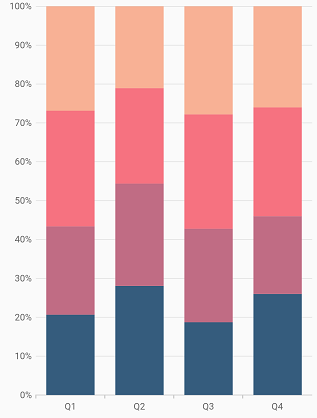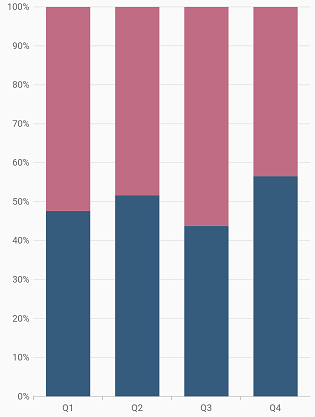# 100% Stacked column in Flutter Cartesian Charts (SfCartesianChart)

11 Apr 202210 minutes to read

To create a Flutter 100% stacked column chart quickly, you can check this video.

To render a 100% stacked column chart, create an instance of `StackedColumn100Series`, and add it to the `series` collection property of `SfCartesianChart`. The following properties can be used to customize the appearance:

``````@override
Widget build(BuildContext context) {
return Scaffold(
body: Center(
child: Container(
child: SfCartesianChart(
primaryXAxis: CategoryAxis(),
series: <ChartSeries>[
StackedColumn100Series<ChartData, String>(
dataSource: chartData,
xValueMapper: (ChartData data, _) => data.x,
yValueMapper: (ChartData data, _) => data.y
),
StackedColumn100Series<ChartData, String>(
dataSource: chartData,
xValueMapper: (ChartData data, _) => data.x,
yValueMapper: (ChartData data, _) => data.y2
),
StackedColumn100Series<ChartData, String>(
dataSource: chartData,
xValueMapper: (ChartData data, _) => data.x,
yValueMapper: (ChartData data, _) => data.y3
),
StackedColumn100Series<ChartData, String>(
dataSource: chartData,
xValueMapper: (ChartData data, _) => data.x,
yValueMapper: (ChartData data, _) => data.y4
)
]
)
)
)
);
}``````## Column width and spacing

The `spacing` property is used to change the spacing between two segments. The default value of spacing is 0, and the value ranges from 0 to 1. Here, 1 and 0 correspond to 100% and 0% of the available space, respectively.

The `width` property is used to change the width of the rectangle. The default value of the width is 0.7, and the value ranges from 0 to 1. Here, 1 and 0 correspond to 100% and 0% of the available width, respectively.

``````@override
Widget build(BuildContext context) {
return Scaffold(
body: Center(
child: Container(
child: SfCartesianChart(
primaryXAxis: CategoryAxis(),
series: <ChartSeries>[
StackedColumn100Series<ChartData, String>(
dataSource: chartData,
xValueMapper: (ChartData data, _) => data.x,
yValueMapper: (ChartData data, _) => data.y,
width: 0.8, // Width of the columns
spacing: 0.2 // Spacing between the columns
),
StackedColumn100Series<ChartData, String>(
dataSource: chartData,
xValueMapper: (ChartData data, _) => data.x,
yValueMapper: (ChartData data, _) => data.y2,
width: 0.8, // Width of the columns
spacing: 0.2 // Spacing between the columns
)
]
)
)
)
);
}``````